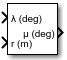# Geocentric to Geodetic Latitude

Convert geocentric latitude to geodetic latitude

• Library:
• Aerospace Blockset / Utilities / Axes Transformations

•## Description

The Geocentric to Geodetic Latitude block converts a geocentric latitude (λ) into geodetic latitude (μ). The function uses an iteration-method of Bowring's formula is used to calculate the geodetic latitude. For more information, see Algorithms.

## Limitations

This implementation generates a geodetic latitude that lies between ±90 degrees.

## Ports

### Input

expand all

Geocentric latitude, specified as a scalar, in degrees. Latitude values can be any value. However, values of +90 and -90 may return unexpected values because of singularity at the poles.

Data Types: `double`

Radius from center of the planet to the center of gravity, specified as a scalar.

Data Types: `double`

### Output

expand all

Geodetic latitude, specified as a scalar, in degrees.

Data Types: `double`

Mean sea-level altitude (MSL), returned as a scalar.

#### Dependencies

To enable this port, select Output altitude.

Data Types: `double`

## Parameters

expand all

Parameter and output units:

Units

Radius from CG to Center of Planet

`Metric (MKS)`

Meters

Meters

`English`

Feet

Feet

#### Programmatic Use

 Block Parameter: `units` Type: character vector Values: ```'Metric (MKS)'``` | `'English'` Default: ```'Metric (MKS)'```

Planet model to use, `Custom` or `Earth (WGS84)`.

#### Programmatic Use

 Block Parameter: `ptype` Type: character vector Values: ```'Earth (WGS84)'``` | `'Custom'` Default: ```'Earth (WGS84)'```

Flattening of the planet, specified as a double scalar.

#### Dependencies

This parameter is enabled when Planet model is set to `Custom`.

#### Programmatic Use

 Block Parameter: `F` Type: character vector Values: double scalar Default: `1/298.257223563`

Radius of the planet at its equator, in the same units as the Units parameter.

#### Dependencies

This parameter is enabled when Planet model is set to `Custom`.

#### Programmatic Use

 Block Parameter: `R` Type: character vector Values: double scalar Default: `6378137`

Select this check box to output the mean sea-level altitude (MSL).

#### Dependencies

Select this check box to enable the h port.

#### Programmatic Use

 Block Parameter: `outputAltitude` Type: character vector Values: `off` | `on` Default: `'off'`

## Algorithms

The Geocentric to Geodetic Latitude block converts a geocentric latitude (λ) into geodetic latitude (μ), where:

• λ — Geocentric latitude

• μ — Geodetic latitude

• r — Radius from the center of the planet

• f — Flattening

• a — Equatorial radius of the plant (semi-major axis)

Given geocentric latitude (λ) and the radius (r) from the center of the planet, this block first converts the desired points into the distance from the polar axis (ρ) and the distance from the equatorial axis (z).

`$\begin{array}{l}\rho =r\left(\mathrm{cos}\left(\lambda \right)\right)\\ z=r\left(\mathrm{sin}\left(\lambda \right)\right).\end{array}$`

It then calculates the geometric properties of the planet:

`$\begin{array}{l}b=a\left(1-f\right)\\ {e}^{2}=f\left(2-f\right)\\ e{\text{'}}^{2}=\frac{{e}^{2}}{\left(1-{e}^{2}\right)}.\end{array}$`

And then uses the fixed-point iteration of Bowring's formula to calculate μ. This formula typically converges in three iterations.

`$\begin{array}{l}\beta ={\mathrm{tan}}^{-1}\left(\frac{\left(1-f\right)\mathrm{sin}\left(\mu \right)}{\mathrm{cos}\left(\mu \right)}\right)\\ \mu ={\mathrm{tan}}^{-1}\left(\frac{z+b{e}^{{\text{'}}^{2}}\mathrm{sin}{\left(\beta \right)}^{3}}{\rho -a{e}^{2}\mathrm{cos}{\left(\beta \right)}^{3}}\right).\end{array}$`

 Jackson, E. B., Manual for a Workstation-based Generic Flight Simulation Program (LaRCsim) Version 1.4, NASA TM 110164, April, 1995.

 Hedgley, D. R., Jr. "An Exact Transformation from Geocentric to Geodetic Coordinates for Nonzero Altitudes." NASA TR R-458, March, 1976.

 Clynch, J. R. "Radius of the Earth - Radii Used in Geodesy." Naval Postgraduate School, Monterey, California, 2002.

 Stevens, B. L., and F. L. Lewis. Aircraft Control and Simulation, Hoboken, NJ: John Wiley & Sons, 1992.

 Edwards, C. H., and D. E. Penny. Calculus and Analytical Geometry 2nd Edition, Prentice-Hall, Englewood Cliffs, New Jersey, 1986.

## Support평가판 신청